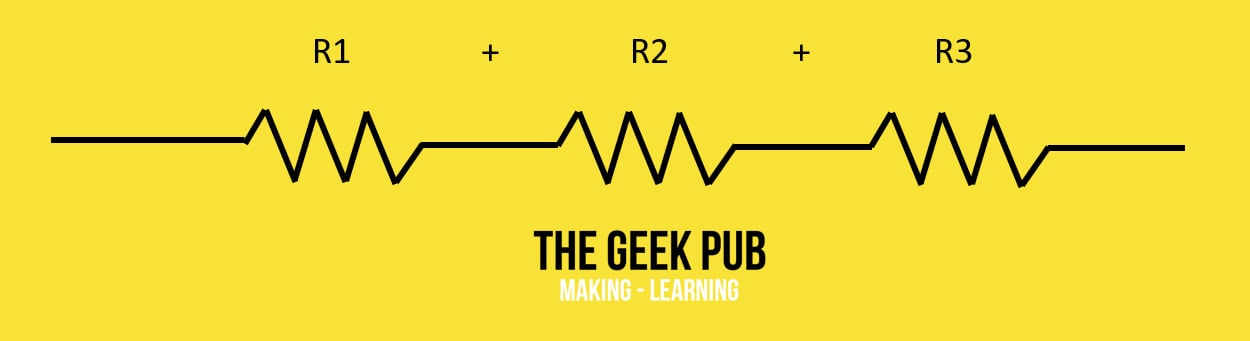When putting resistors in series the total resistance value needs to be calculated.  This handy resistor calculator will help you do that.

## Series Resistor Calculator

 No of Resistors: 2 3 4 5 6 7 8 9 10 11 12 13 14 15

 Resistor 1 OhmKilo OhmMega Ohm Resistor 2 OhmKilo OhmMega Ohm

### Output Value

In a series circuit the same amount of current passes through all the resistors. This means the total resistance is equal to the sum of all of the resistors added together.  The series resistor calculator sums the value of each resistor and outputs a total resistance value.The formula for calculating series resistance is: R = R1 + R2 + R3 + R4 + Rn

Rn is for extrapolation.  If you have more than 4 resistors, just keep adding them up by changing Rn to R4, R5, etc.

You might enjoy our resistor tutorial as well.  We also have an LED resistor calculator, and a resistor color code calculator.